www.batmath.it

### Discontinuity

A function that is not continuous at a point c of its domain is called discontinuous at c. Usually this concept of discontinuity is used also in a larger sense:  if a point c is an accumulation point of the domain that does not belong to the domain, then the function is called discontinuous at c. For example the function f(x)=lnx is called discontinuous at zero. But pay attention because, as zero does not belong to the domain, it makes no sense to ask whether the functions is continuous or not at zero. So, strictly speaking, the function is neither continuous not discontinuous at zero: the word discontinuous is used here in a somewhat improper sense.

A function may be discontinuous at a point c (that belongs to the domain or is an accumulation point for the domain) for one of the following reasons:

•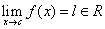, but lf(c). In this case the function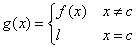is almost the same than f and is continuous at c: it is called a continuous extension of f. The discontinuity is removable.

•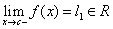and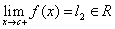, but l1l2. In this case we'll say that function jumps between l1 and l2 and |l1-l2| is the height of the jump.

•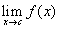does not exist or is infinite.

Examples

• Consider the function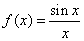. As zero does not belong to the domain but is an accumulation point for the domain, the function is discontinuous at zero. The function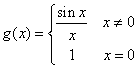is continuous everywhere and is a continuous extension of f.

• Consider the function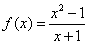. As -1 does not belong to the domain but is an accumulation point for the domain, the function is discontinuous at -1. The function g(x)=x-1 is a continuous extension of f.

• Consider the function f(x)=|sign(x)|. The function is not continuous at zero because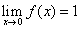while f(0)=0. The constant function f(x)=1 is a continuous extension of f.

• Consider the function f(x)=sign(x). As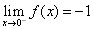and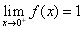, the function has a jump at zero with height two.

• Consider the function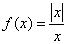. Asand, the function has a jump at zero with height two. Observe that, unlike the previous example, zero does not belong to the domain.

• Consider the function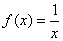. As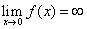the function is discontinuous at zero (observe that zero does not belong to the domain of the function).

• Consider the function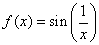. As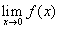does not exist, the function is discontinuous at zero.

first published on march 26 2002 - last updated on september 01 2003